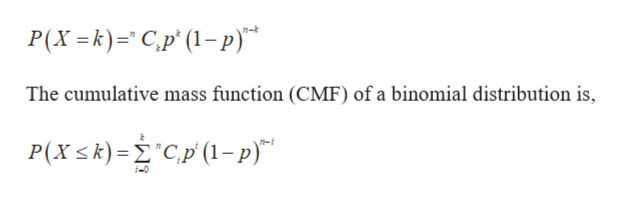# An optical inspection system is used to distinguish among different part types. The probability of correct classification of any part is 0.98. Suppose that three parts are inspected and that the classifications are independent. Let the random variable X denote the number of parts that are correctly classified. Determine the probability mass function and cumulative mass function of X.

Question

An optical inspection system is used to distinguish among different part types. The probability of correct classification of any part is 0.98. Suppose that three parts are inspected and that the classifications are independent. Let the random variable X denote the number of parts that are correctly classified. Determine the probability mass function and cumulative mass function of X.

check_circleExpert Solution
Step 1

Here, X is a random variable that denotes the number of parts that are correctly classified.

The number of parts inspected (n) is 3.

The probability of correct classification of a single part is 0.98.

It is provided that the classifi...help_outlineImage TranscriptioncloseP(X =k)C,p' (1-p) The cumulative mass function (CMF) of a binomial distribution is, P(Xk)=Cp (1-p)" fullscreen

### Want to see the full answer?

See Solution

#### Want to see this answer and more?

Solutions are written by subject experts who are available 24/7. Questions are typically answered within 1 hour*

See Solution
*Response times may vary by subject and question
Tagged in

### Other